# NCERT Solutions For Class 10 Maths Chapter 3 Exercise 3.4 – Pair of Linear Equations in two Variables

Download NCERT Solutions For Class 10 Maths Chapter 3 Exercise 3.4 – Pair of Linear Equations in two Variables. This Exercise contains 2 questions, for which detailed answers have been provided in this note. In case you are looking at studying the remaining Exercise for Class 10 for Maths NCERT solutions for other Chapters, you can click the link at the end of this Note.

### NCERT Solutions For Class 10 Maths Chapter 3 Exercise 3.4 – Pair of Linear Equations in two Variables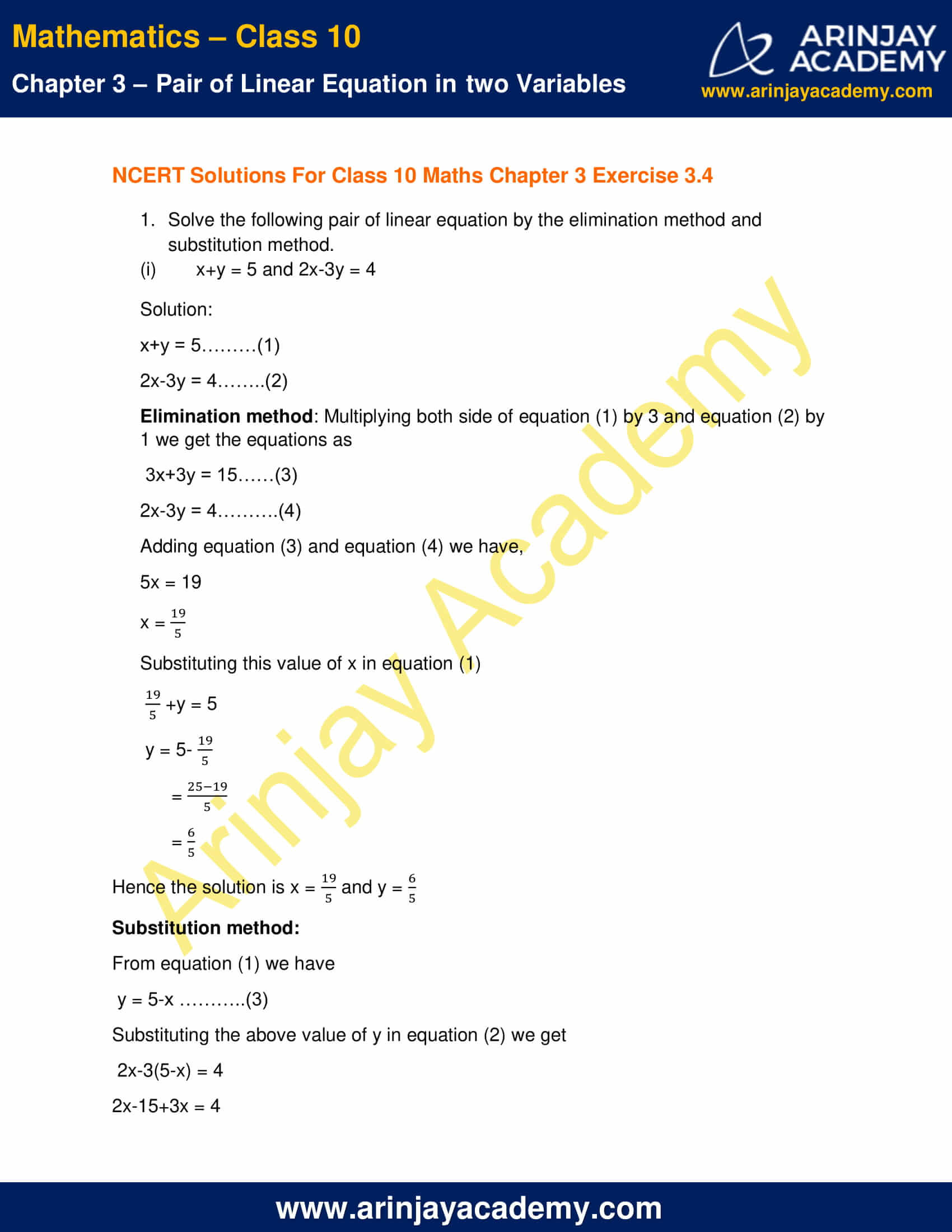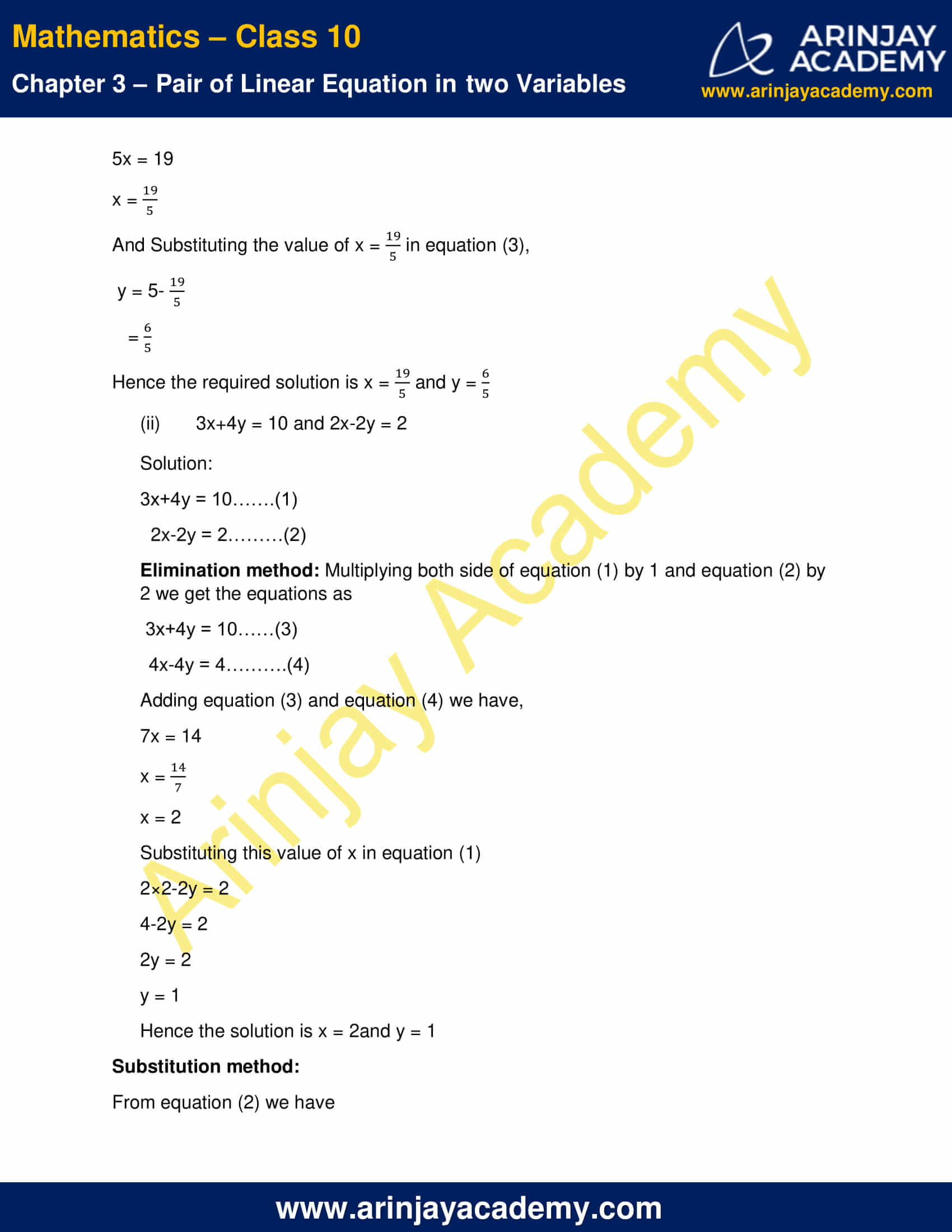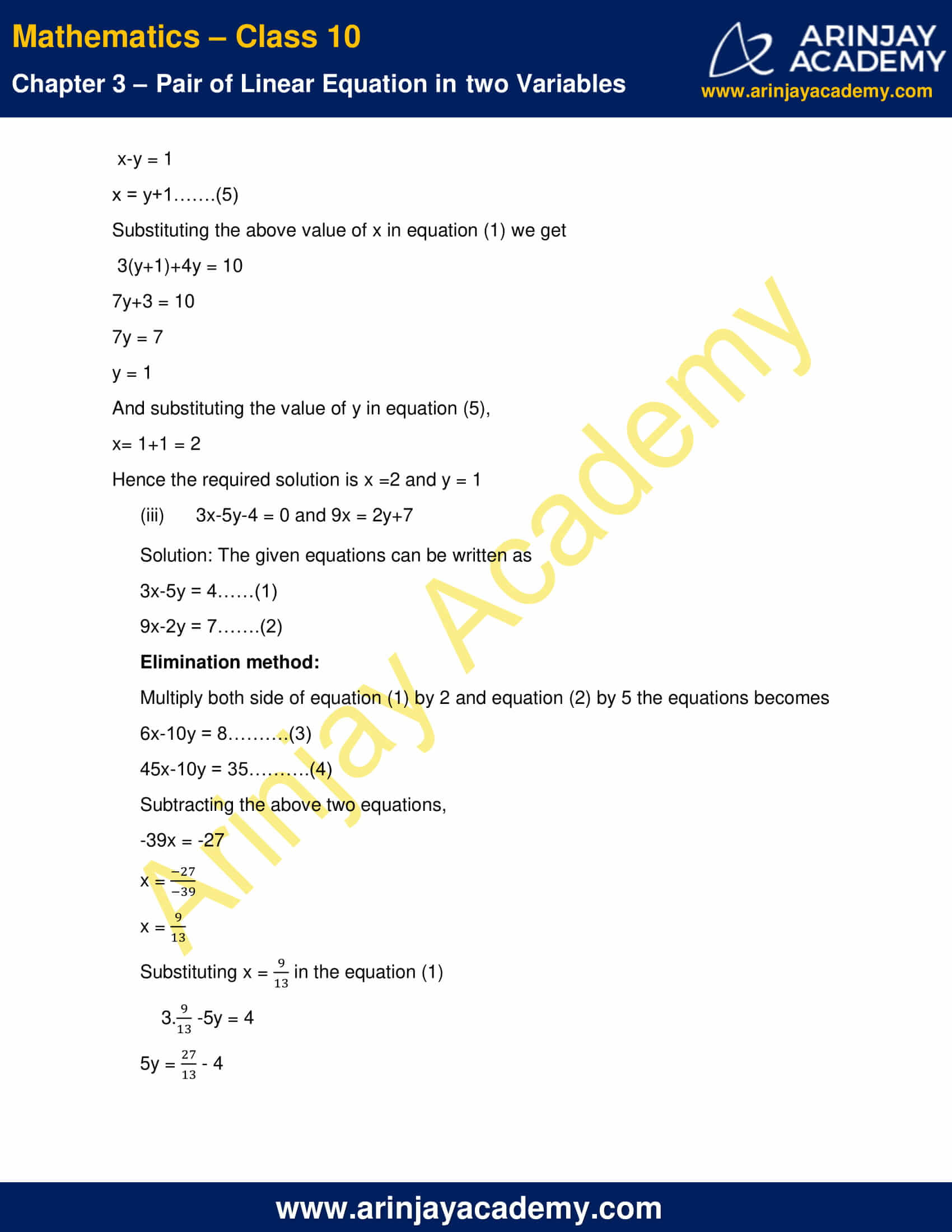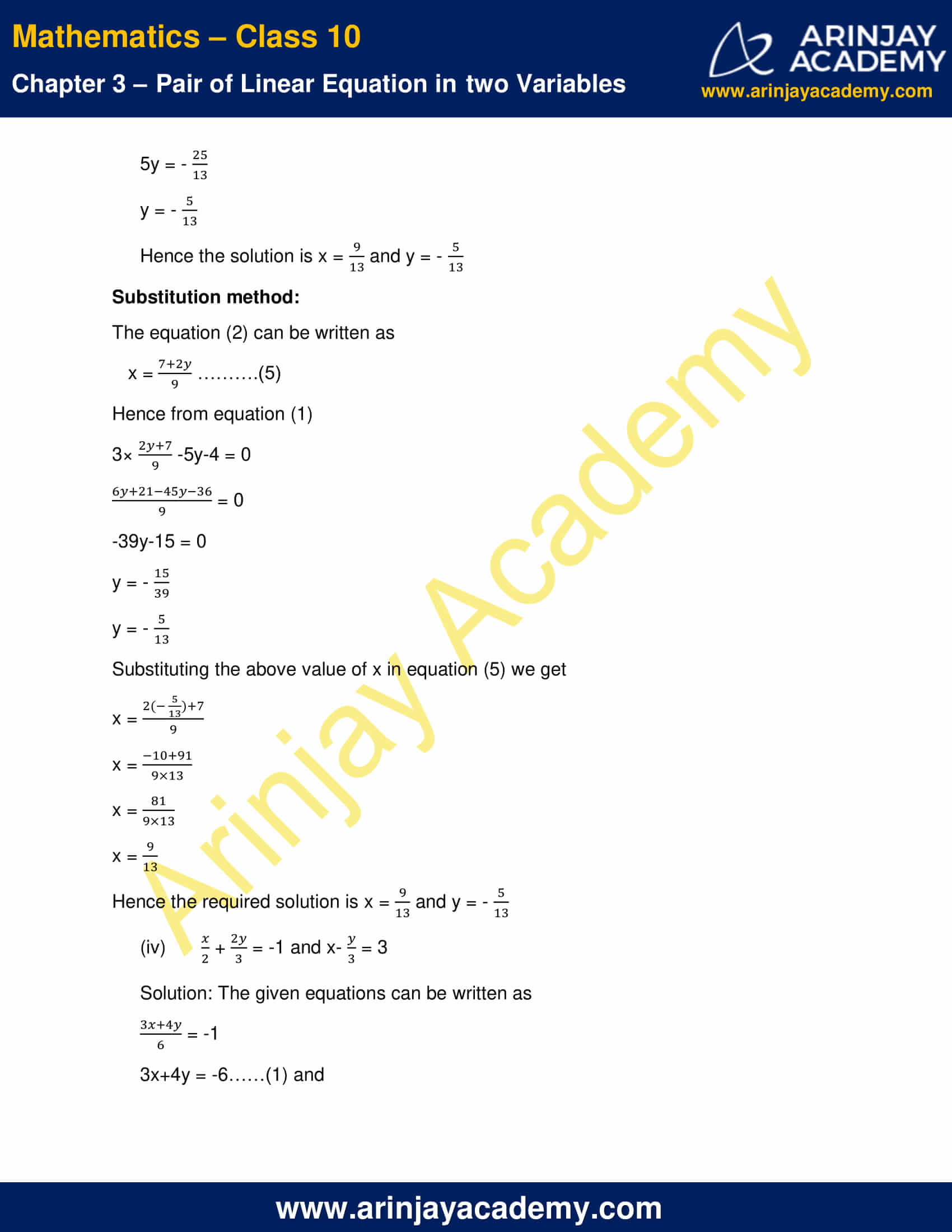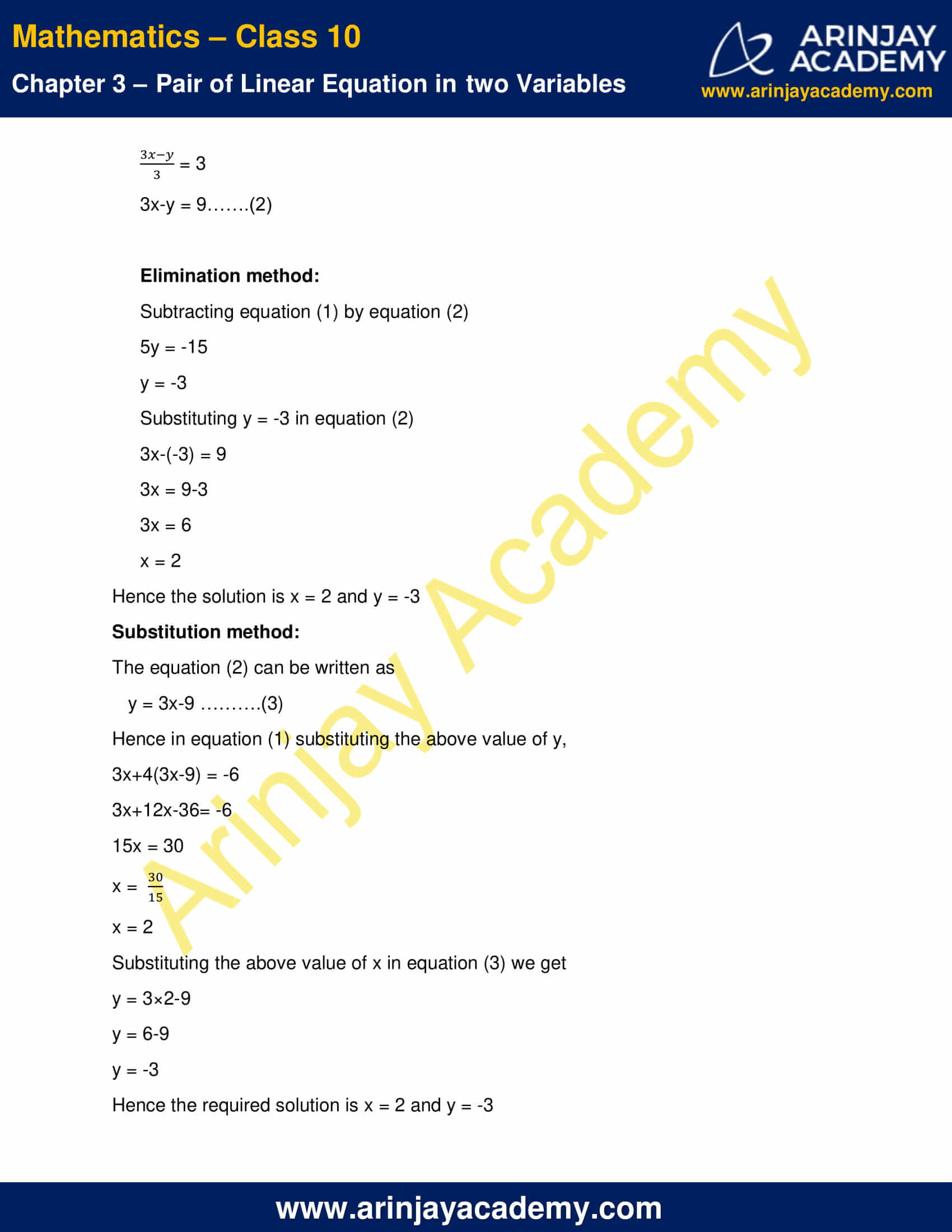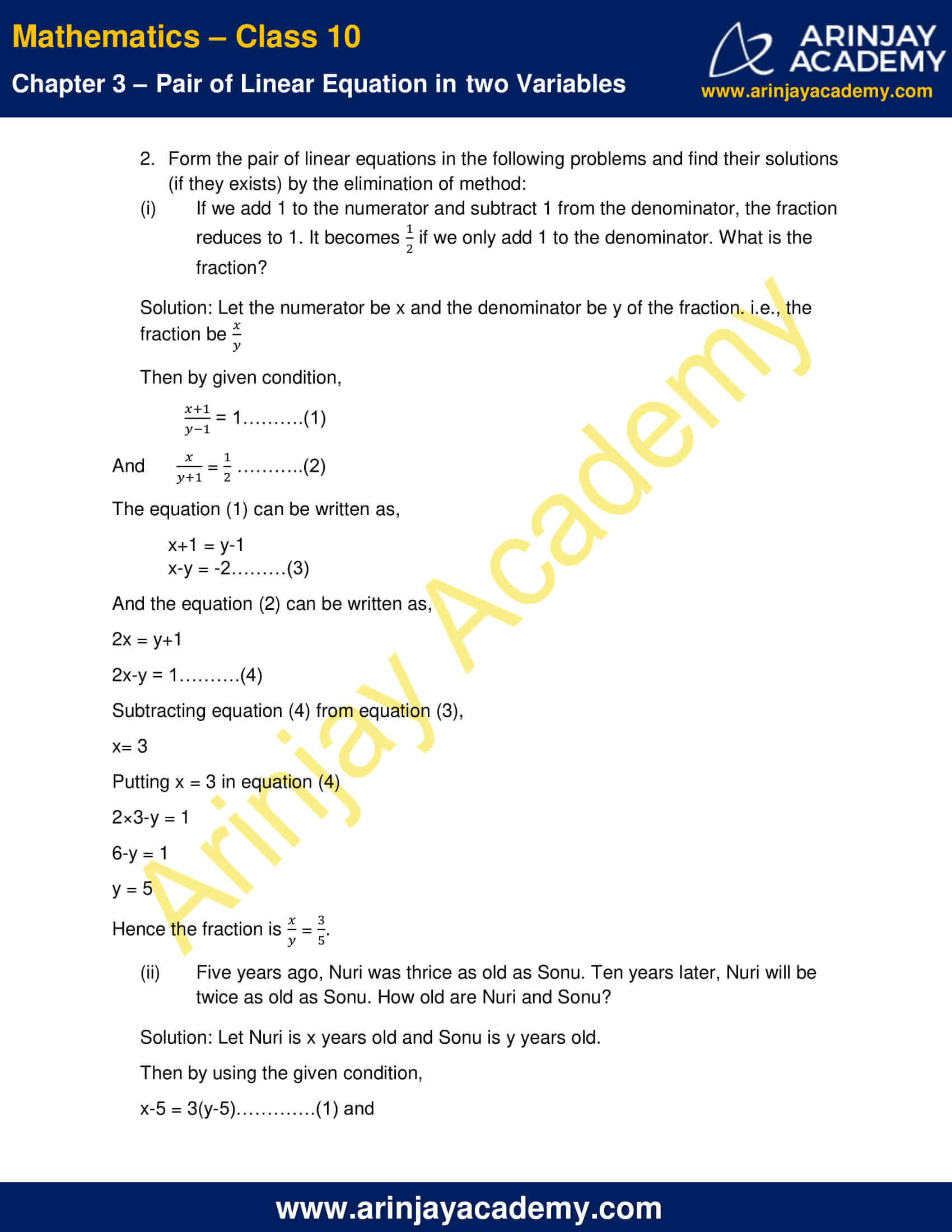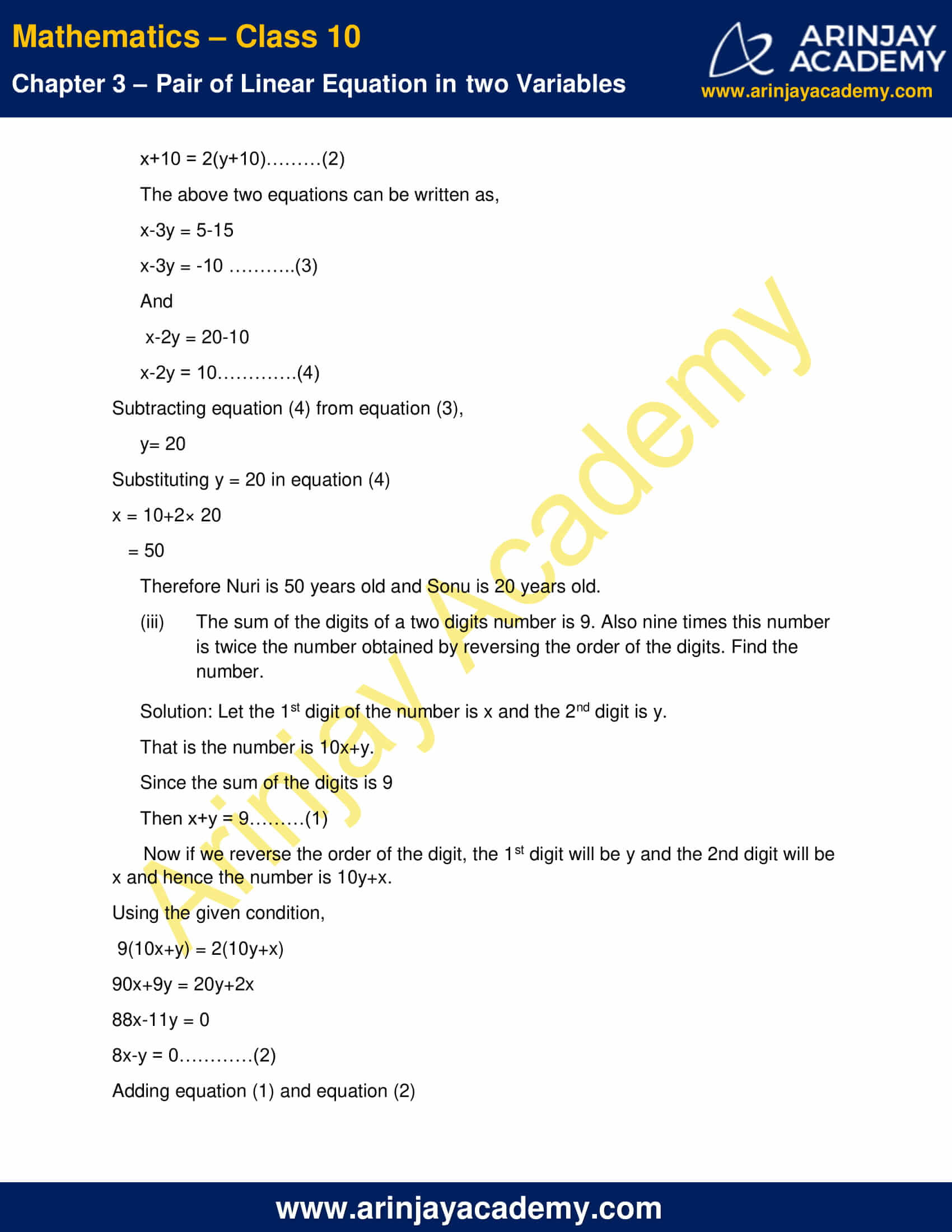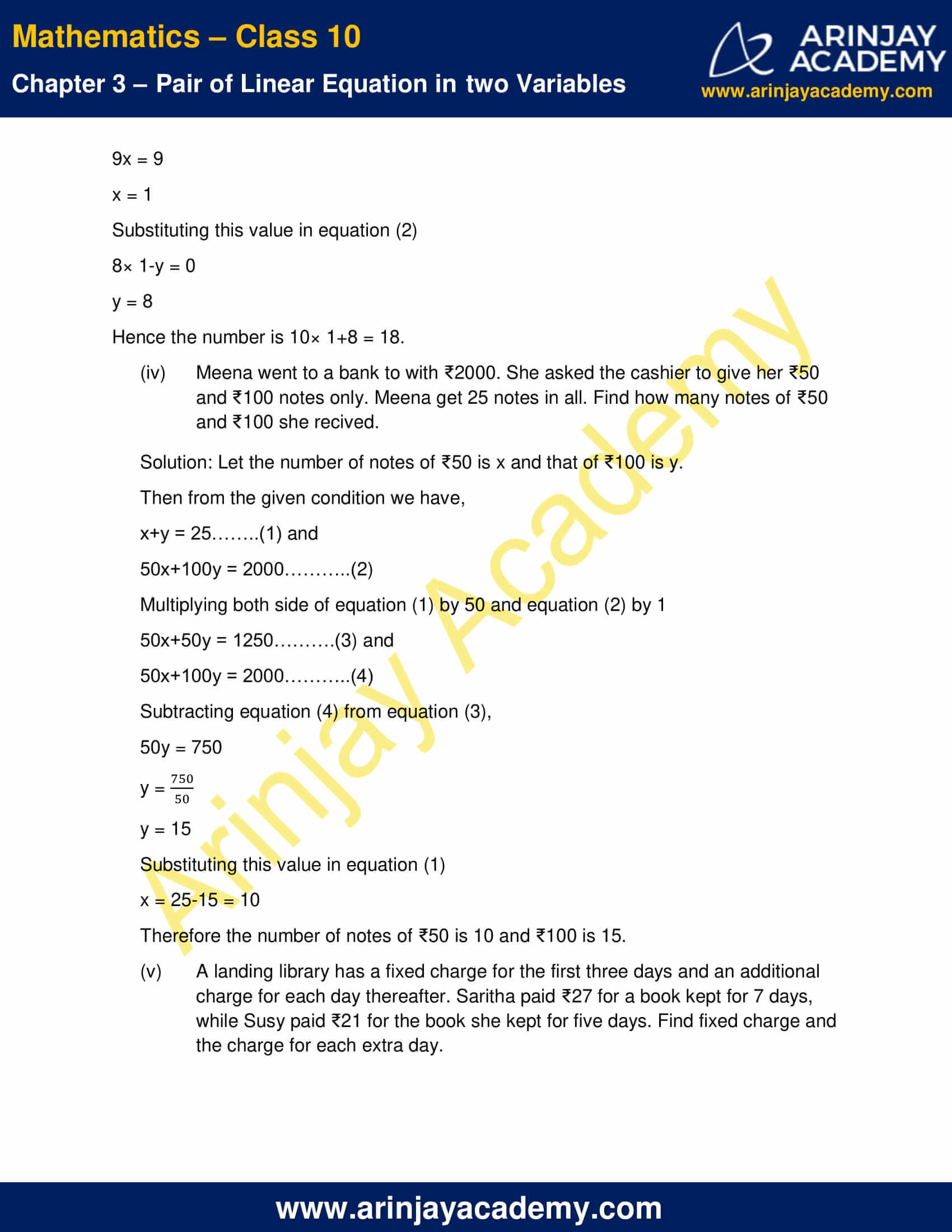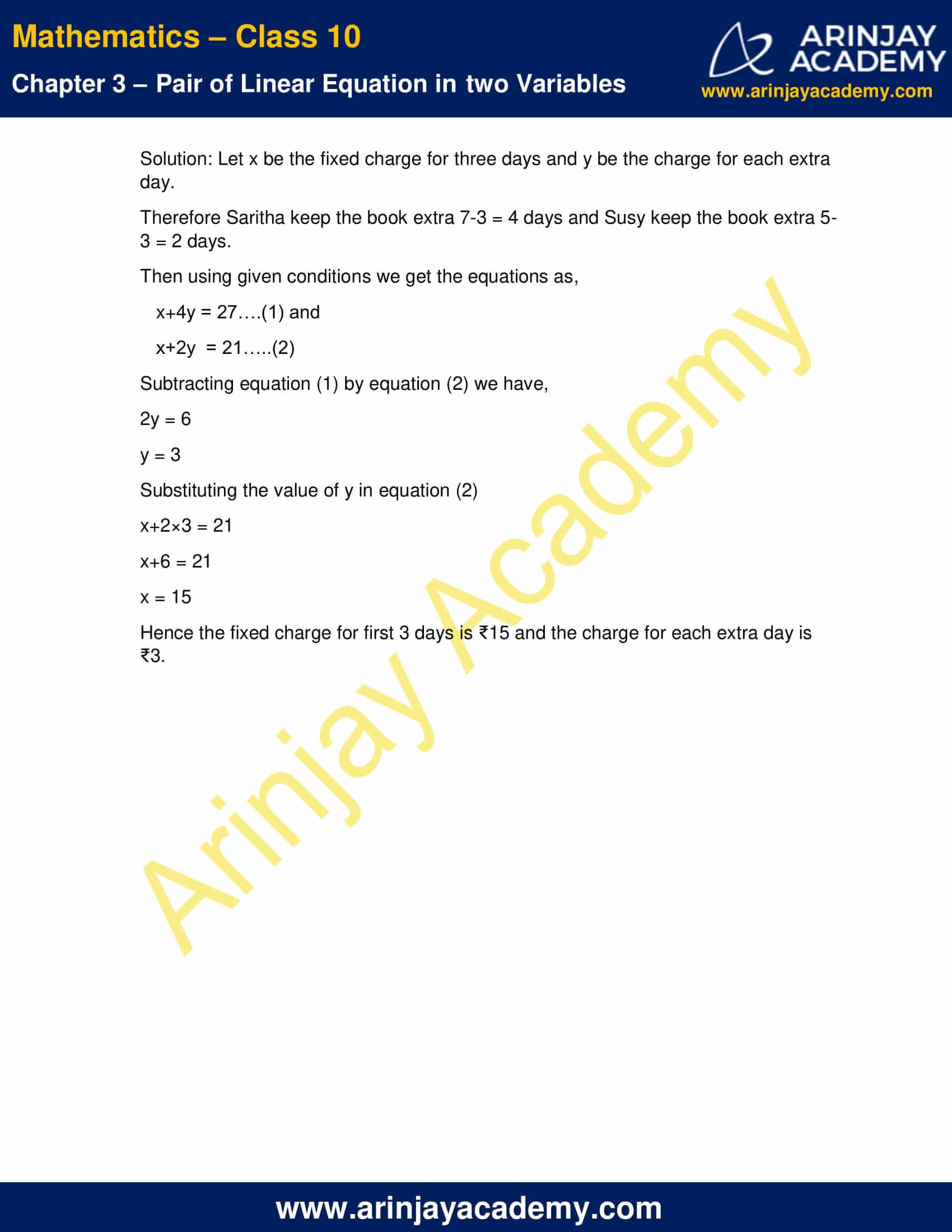NCERT Solutions For Class 10 Maths Chapter 3 Exercise 3.4 – Pair of Linear Equations in two Variables

1. Solve the following pair of linear equation by the elimination method and substitution method.

(i) x+y = 5 and 2x-3y = 4

Solution:

x+y = 5………(1)

2x-3y = 4……..(2)

Elimination method: Multiplying both side of equation (1) by 3 and equation (2) by 1 we get the equations as

3x+3y = 15……(3)

2x-3y = 4……….(4)

Adding equation (3) and equation (4) we have,

5x = 19

x = 19/5

Substituting this value of x in equation (1)

(19/5)+y = 5

y = 5-(19/5)

= (25-19/5)

= (6/5)

Hence the solution is x = (19/5) and y = (6/5)

Substitution method:

From equation (1) we have

y = 5-x ………..(3)

Substituting the above value of y in equation (2) we get

2x-3(5-x) = 4

2x-15+3x = 4

5x = 19

x = (19/5)

And Substituting the value of x = (19/5) in equation (3),

y = 5-(19/5)

= 6/5

Hence the required solution is x = (19/5) and y = (6/5)

(ii) 3x+4y = 10 and 2x-2y = 2

Solution:

3x+4y = 10…….(1)

2x-2y = 2………(2)

Elimination method: Multiplying both side of equation (1) by 1 and equation (2) by 2 we get the equations as

3x+4y = 10……(3)

4x-4y = 4……….(4)

Adding equation (3) and equation (4) we have,

7x = 14

x = 14/7

x = 2

Substituting this value of x in equation (1)

2×2-2y = 2

4-2y = 2

2y = 2

y = 1

Hence the solution is x = 2 and y = 1

Substitution method:

From equation (2) we have

x-y = 1

x = y+1…….(5)

Substituting the above value of x in equation (1) we get

3(y+1)+4y = 10

7y+3 = 10

7y = 7

y = 1

And substituting the value of y in equation (5),

x= 1+1 = 2

Hence the required solution is x =2 and y = 1

(iii) 3x-5y-4 = 0 and 9x = 2y+7

Solution: The given equations can be written as

3x-5y = 4……(1)

9x-2y = 7…….(2)

Elimination method:

Multiply both side of equation (1) by 2 and equation (2) by 5 the equations becomes

6x-10y = 8……….(3)

45x-10y = 35……….(4)

Subtracting the above two equations,

-39x = -27

x = (-27/-39)

x = 9/13

Substituting x =9/13 in the equation (1)

3.(9/13) – 5y = 4

5y = (27/13) – 4

5y = -(25/13)

y = -(5/13)

Hence the solution is x = (9/13) and y = -(5/13)

Substitution method:

The equation (2) can be written as

x = (7+2y/9) …….(5)

Hence from equation (1)

3×(2y+7/9) -5y-4 = 0

(6y+21-45y-36/9)= 0

-39y-15 = 0

y = -(15/39)

y = -(5/13)

Substituting the above value of x in equation (5) we get

x = 2[(-5/13)+7/9]

x = (-10+91/9 x 13)

x = (81/9 x 13)

x = (9/13)

Hence the required solution is x = (9/13) and y = -(5/13)

(iv) (x/2) + (2y/3) = -1 and x-(y/3)  = 3

Solution: The given equations can be written as

(3x+4y/6) = -1

3x+4y = -6……(1) and

(3x-y/3) = 3

3x-y = 9…….(2)

Elimination method:

Subtracting equation (1) by equation (2)

5y = -15

y = -3

Substituting y = -3 in equation (2)

3x-(-3) = 9

3x = 9-3

3x = 6

x = 2

Hence the solution is x = 2 and y = -3

Substitution method:

The equation (2) can be written as

y = 3x-9 ……….(3)

Hence in equation (1) substituting the above value of y,

3x+4(3x-9) = -6

3x+12x-36= -6

15x = 30

x = (30/15)

x = 2

Substituting the above value of x in equation (3) we get

y = 3×2-9

y = 6-9

y = -3

Hence the required solution is x = 2 and y = -3

2. Form the pair of linear equations in the following problems and find their solutions (if they exists) by the elimination of method:

(i) If we add 1 to the numerator and subtract 1 from the denominator, the fraction reduces to 1. It becomes if we only add 1 to the denominator. What is the fraction?

Solution: Let the numerator be x and the denominator be y of the fraction. i.e., the fraction be x/y

Then by given condition,

(x+1/y-1) = 1……….(1)

And  (x/y+1) = (1/2) ………..(2)

The equation (1) can be written as,

x+1 = y-1

x-y = -2………(3)

And the equation (2) can be written as,

2x = y+1

2x-y = 1……….(4)

Subtracting equation (4) from equation (3),

x= 3

Putting x = 3 in equation (4)

2×3-y = 1

6-y = 1

y = 5

Hence the fraction is (x/y) = (3/5).

(ii) Five years ago, Nuri was thrice as old as Sonu. Ten years later, Nuri will be twice as old as Sonu. How old are Nuri and Sonu?

Solution: Let Nuri is x years old and Sonu is y years old.

Then by using the given condition,

x-5 = 3(y-5)………….(1) and

x+10 = 2(y+10)………(2)

The above two equations can be written as,

x-3y = 5-15

x-3y = -10 ………..(3)

And

x-2y = 20-10

x-2y = 10………….(4)

Subtracting equation (4) from equation (3),

y= 20

Substituting y = 20 in equation (4)

x = 10+2× 20

= 50

Therefore Nuri is 50 years old and Sonu is 20 years old.

(iii) The sum of the digits of a two digits number is 9. Also nine times this number is twice the number obtained by reversing the order of the digits. Find the number.

Solution: Let the 1st digit of the number is x and the 2nd digit is y.

That is the number is 10x+y.

Since the sum of the digits is 9

Then x+y = 9………(1)

Now if we reverse the order of the digit, the 1st digit will be y and the 2nd digit will be x and hence the number is 10y+x.

Using the given condition,

9(10x+y) = 2(10y+x)

90x+9y = 20y+2x

88x-11y = 0

8x-y = 0…………(2)

Adding equation (1) and equation (2)

9x = 9

x = 1

Substituting this value in equation (2)

8× 1-y = 0

y = 8

Hence the number is 10× 1+8 = 18.

(iv) Meena went to a bank to with ₹ She asked the cashier to give her ₹50 and ₹100 notes only. Meena get 25 notes in all. Find how many notes of ₹50 and ₹100 she recived.

Solution: Let the number of notes of ₹50 is x and that of ₹100 is y.

Then from the given condition we have,

x+y = 25……..(1) and

50x+100y = 2000………..(2)

Multiplying both side of equation (1) by 50 and equation (2) by 1

50x+50y = 1250……….(3) and

50x+100y = 2000………..(4)

Subtracting equation (4) from equation (3),

50y = 750

y = 750/50

y = 15

Substituting this value in equation (1)

x = 25-15 = 10

Therefore the number of notes of ₹50 is 10 and ₹100 is 15.

(v) A landing library has a fixed charge for the first three days and an additional charge for each day thereafter. Saritha paid ₹27 for a book kept for 7 days, while Susy paid ₹21 for the book she kept for five days. Find fixed charge and the charge for each extra day.

Solution: Let x be the fixed charge for three days and y be the charge for each extra day.

Therefore Saritha keep the book extra 7-3 = 4 days and Susy keep the book extra 5-3 = 2 days.

Then using given conditions we get the equations as,

x+4y = 27….(1) and

x+2y  = 21…..(2)

Subtracting equation (1) by equation (2) we have,

2y = 6

y = 3

Substituting the value of y in equation (2)

x+2×3 = 21

x+6 = 21

x = 15

Hence the fixed charge for first 3 days is ₹15 and the charge for each extra day is ₹3.

NCERT Solutions for Class 10 Maths Chapter 3 Exercise 3.4 – Pair of Linear Equations in two Variables, has been designed by the NCERT to test the knowledge of the student on the topic – Algebraic Methods of Solving a Pair of Linear Equations – Elimination Method

Download NCERT Solutions For Class 10 Maths Chapter 3 Exercise 3.4 – Pair of Linear Equations in two Variables